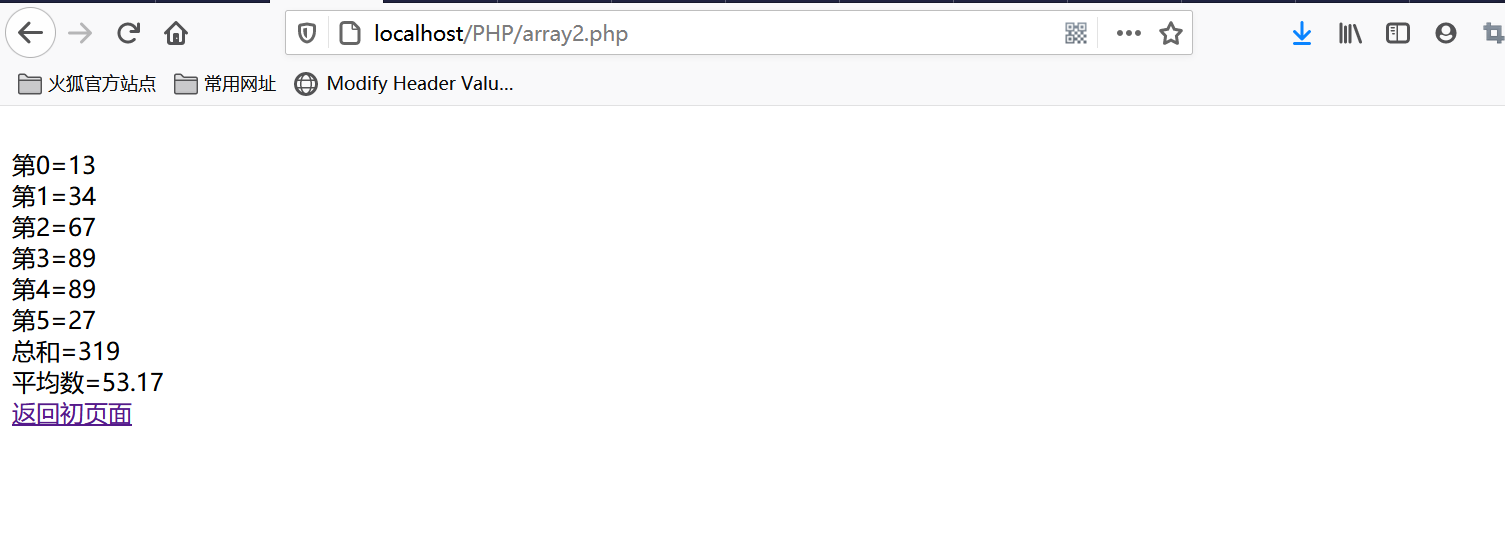PHP入门之类型与运算符
PHP入门之流程控制
PHP入门之函数

# 数组初探

<?php
$sums=10;$sums=11;
$sums=12.6;$sums=24;
$sums=56;$sums=28;
$allsums=0; #count系统函数是用来统计数组个数的。 for ($i=0;$i<count($sums);$i++){ #这里使用for循环遍历数组，也可以while循环和do while 循环，都可以。 echo '<br/>'.$sums[$i]; # 但是使用这三个循环遍历，键值不能改，默认的0、1、2等等，只能是数字$allsums+=$sums[$i];             # 如果想自定义键值，那么就要使用foreach()来遍历数组。
}
echo '<br/>'.$allsums; #求总数 echo '<br/>'.$allsums/count($sums); #求平均数 ?>  理清楚几个概念，用$sums=10来举例
 这个我们叫下标或者关键字。
$sums 这个我们叫做数组的一个元素。 10 这个我们叫做$sums对应的值。
$sums 这个叫做数组的名称。 还有就是元素存放的值可以是任何数据类型。 举个例子 <?php$arr=123;
$arr=34.789;$arr="hello";

for($i=0;$i<count($arr);$i++){       #可以正常输出 ： 123 34.789  hello

echo '<br/>'.$arr[$i];
}
?>


<?php
$arr=array(123,34.789,"hello"); for ($i=0;$i<count($arr);$i++){ echo '<br/>'.$arr[$i]; #可以正常输出 ： 123 34.789 hello } ?>  第三种方法创建数组的方法 举个例子： $arr['logo']="123";
$arr['haha']=34.789;$arr['qqq']="hello";


$arr=array("logo"=>123,"haha"=>34.789,"qqq"=>"hello"); 这样的话遍历就用foreach()用来遍历。 代码如下 foreach ($arr as $key=>$val){
echo "<br/>$key=$val";
}


$arr=array("logo"=>123,"haha"=>34.789,"qqq"=>"hello");$arr['logo']="lxf";
foreach ($arr as$key=>$val){ echo "<br/>$key=$val"; } echo '<br/>'.$arr['logo'];


Array ( [logo] => lxf [haha] => 34.789 [qqq] => hello )
array(3) { ["logo"]=> string(3) "lxf" ["haha"]=> float(34.789) ["qqq"]=> string(5) "hello" }

php语言中，数组可以动态增长，举个例子。

$arr=array(4,7,9);$arr=466;
echo $arr;  在这个例子中，会输出466，不会报错。 与PHP数组相关的几个重要函数 （1）count函数 统计数组元素个数 （2）is_array函数 用来确定是不是数组，举个例子。 $arr=array(12,34,24);
echo is_array($arr); #这里会返回1为真。如果$arr=123;
echo is_array($arr); #这里会返回空，为假。  （3）拆分字符串explode("按照什么拆分"，字符串)，举个例子 $str="老大,老二,老三,老四";
$arr=explode(",",$str);
print_r($arr);  输出结果为： #由字符串变为数组了。 Array (  => 老大  => 老二  => 老三  => 老四 )  如果要删除某个元素的键-值对需要使用unset()函数，需要注意的是，删除后不会重新建立索引。举个例子： $arr=array(1,2,5,8);
unset($arr); print_r($arr);


unset也可以销毁某个变量
Array (  => 1  => 2  => 8 )

$arr=array(1,2,5,8); unset($arr,$arr); print_r($arr);


Array (  => 1  => 2 )

$a +$b 联合 $a 和$b 的联合。
$a ==$b 相等 如果 $a 和$b 具有相同的键／值对则为 TRUE。
$a ===$b 全等 如果 $a 和$b 具有相同的键／值对并且顺序和类型都相同则为 TRUE。
$a !=$b 不等 如果 $a 不等于$b 则为 TRUE。
$a <>$b 不等 如果 $a 不等于$b 则为 TRUE。
$a !==$b 不全等 如果 $a 不全等于$b 则为 TRUE。

• 运算符把右边的数组元素附加到左边的数组后面，两个数组中都有的键名，则只用左边数组中的，右边的被忽略。

# 实例

array1.php
<html>
<title>数组练习</title>
<meta http-equiv="content-type" content="text/html;charset=utf-8"/>
<body>
<h1>输入6个数据，空格隔开</h1>
<form action="array2.php" method="post">
<input type="text" name="sum">
<input type="submit" value="提交">
</form>
</body>
</html>


array2.php

<?php
$sums=$_POST['sum'];
$arr=explode(" ",$sums);
$allarr=0; foreach ($arr as $key=>$val){
$allarr+=$val;
#遍历数组
echo '<br/>'."第"."$key"."=".$val;
}
#求总数
echo '<br/>'."总和=".$allarr; #求平均值 echo '<br/>'."平均数=".round($allarr/count(\$arr),2);
?>
<br/><a href="array1.php">返回初页面</a># 小结

posted @ 2020-08-06 00:36  雪痕*  阅读(160)  评论(2编辑  收藏  举报